# VBA TypeName

The VBA TypeName Function is used in determining the type of data stored in a cell, or the type of a selected object – for example a worksheet, range or cell, or a control on a form.

## Determining the Data Type in a Cell

To determine was datatype in in a cell we can use the TypeName function with the Cells Property.

``````Sub TestCellDataType()
MsgBox "The type of data in " & Cells(3, 2).Address & " is " & TypeName(Cells(3, 2).Value)
End Sub``````

If we run this code above with the worksheet below, the message box will tell us what type of data is in the cell.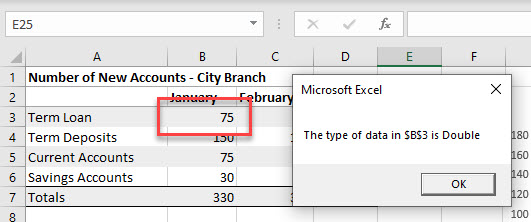## Determining the type of Object Selected

We can also use TypeName to determine what type of Object has been selected in a worksheet – a Range or a Chart for example.

``````Sub TestSelection()
MsgBox "You have selected a " & TypeName(Selection)
End Sub``````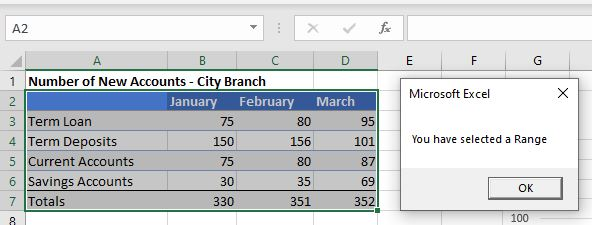Or, if we select a chart: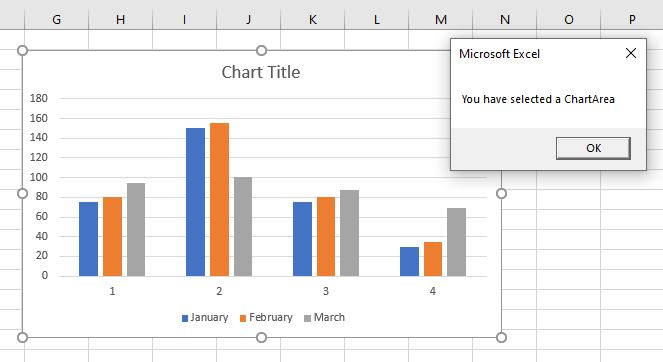We can drill down even further and select the objects within the chart, and the macro will return what we have selected.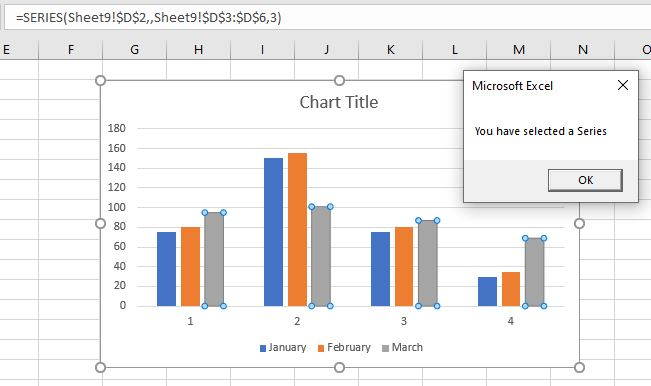All of this can be most useful in building our VBA project to either control the flow of the code, or to prevent errors from occurring by testing to ensure the correct type of object is selected, or the correct type of data is entered into a cell.

## Using TypeName on Form Controls

VBA enables us to create interactive forms that the user can fill in and return data to the code to be used in various ways.  We can use the TypeName operator to determine the type of controls that are being used on a form.

In the example below, I have created a user form with a variety of controls on it – a couple of Text Boxes, a Combo Box, 2 option buttons, 2 check boxes and 3 command buttons.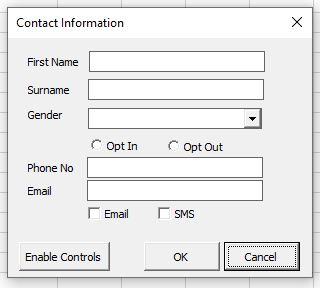Using the code below, I can determine what type of controls are on the form by looping through all the controls on the form. I have used the TypeName function to return a message with the type of the control with a VBA IF Statement to check what type of control is selected.

``````Sub WhatControlType()
Dim ctl As Object
For Each ctl In Me.Controls
MsgBox "The control is a " & TypeName(ctl)
Next ctl
End Sub``````

This type of code can be very useful if we wish to enable or disable controls. In the code below, when the form is first opened, the option buttons and check boxes are disabled.

``````Private Sub UserForm_Initialize()
Dim ctl As Object
For Each ctl In Me.Controls
If TypeName(ctl) = "CheckBox" Then
ctl.Enabled = False
ElseIf TypeName(ctl) = "OptionButton" Then
ctl.Enabled = False
Else
ctl.Enabled = True
End If
Next ctl
End Sub

``````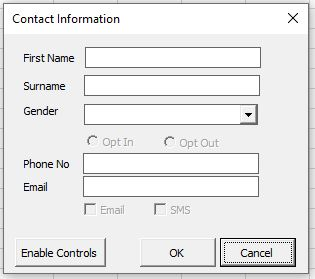To enable the option buttons and checkboxes, I have written some further code behind the Enable Controls button.

``````Private Sub cmdEnable_Click()
Dim ctl As Object
For Each ctl In Me.Controls
If TypeName(ctl) = "CheckBox" Then
ctl.Enabled = Not ctl.Enabled
ElseIf TypeName(ctl) = "OptionButton" Then
ctl.Enabled = Not ctl.Enabled
End If
Next ctl
End Sub``````

The functionality in this code can also be created using the VBA TypeOf Operator.

Stop searching for VBA code online. Learn more about AutoMacro - A VBA Code Builder that allows beginners to code procedures from scratch with minimal coding knowledge and with many time-saving features for all users!